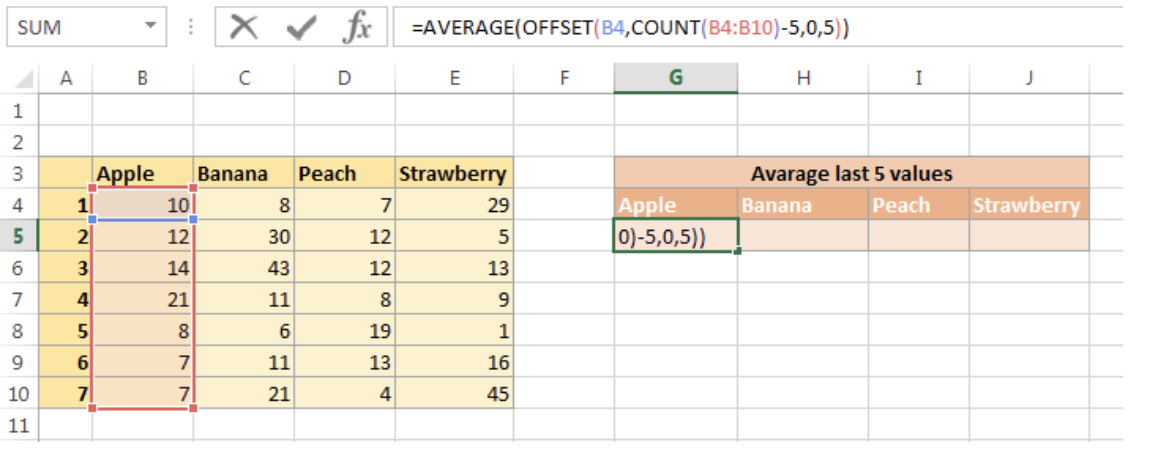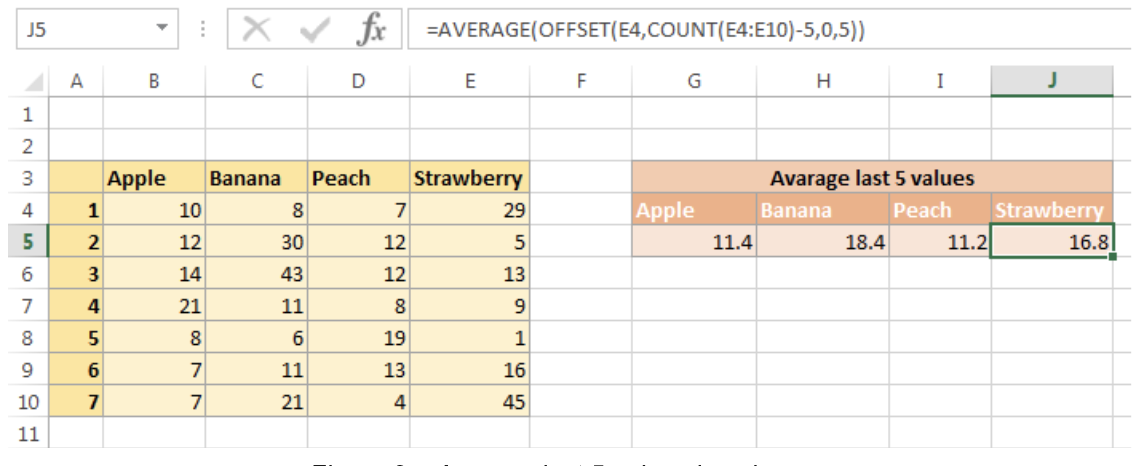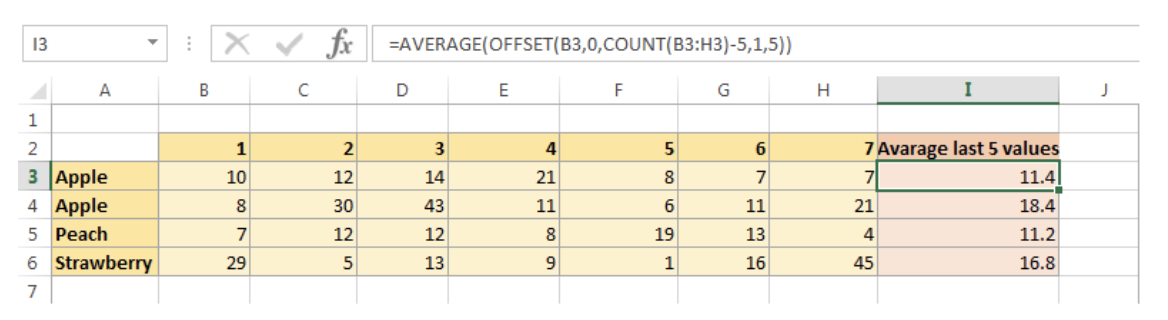Get instant live expert help with Excel or Google Sheets“My Excelchat expert helped me in less than 20 minutes, saving me what would have been 5 hours of work!”

#### Post your problem and you’ll get Expert help in seconds.

Your message must be at least 40 characters
Our professional Expert are available now. Your privacy is guaranteed.

# Average last 5 values in columns

You can use AVERAGE, OFFSET, and COUNT functions to average last 5 values in columns.

Generic Formula

`=AVERAGE(OFFSET(first_cell,COUNT(range)-5,0,5))`

Range: Required. The range that you want to take 5 last values from it

First_cell: Required. The first cell of the range

The OFFSET function is used here to return an array of a range with rows, columns, height, and weight. The AVERAGE function then averages the elements in the array.

## Example:

Considering the example below. The formula in G5 is `=AVERAGE(OFFSET(B4,COUNT(B4:B10)-5,0,5))`Figure 1 – Average last 5 values in columns

## Explanation

OFFSET function has syntax like this: OFFSET(reference, rows, cols, [height], [width])

In this case, this is how `OFFSET(B4`,COUNT`(B4`:B10`)-5,0,5)` portion works:

• Reference: It bases the offset from B4
• Rows: COUNT function counts the number of rows in range B4:B10, which is 7 rows. It then subtracts 5 because we want the offset to go down 2 row from the base, leave the last 5 rows for the returned range.
• Cols: is 0 because we want the offset to stay in the same column
• [height]: is 5 because we want the returned range with 5 rows.
• [width]: is omitted here. It defaults 1, which means the returned range with 1 column.

OFFSET function returns an array like this {14;21;8;7;7}

Finally, AVERAGE function averages the array and returns 11.4

Extending the formula through column G to J to get other results:Figure 2 – Average last 5 values in columns

If your data is in a horizontal arrangement, you can change the formula a little bit to adapt to the same works.Figure 3 – Average last 5 values in columns

OFFSET(B3,0,COUNT(B3:H3)-5,1,5):

• Reference: It bases the offset from B3
• Rows: 0 because we want to stay in the same row
• Cols: COUNT function counts the number of columns in range B3:H3, which is 7 columns. It then subtracts 5 because we want the offset to go right 2 columns from the base, leave the last 5 columns for the returned range
• [height]: is 1 because we want the returned range with 1 rows. It cannot be omitted like the previous case because you need to fill this argument before giving the next argument.
• [width]: is 5 which means the returned range with 5 columns.

If you want to average N last values, replace number 5 with number N in the main formula.

Are you still looking for help with the Average function? View our comprehensive round-up of Average function tutorials here.

### Did this post not answer your question? Get a solution from connecting with the expert.Another blog reader asked this question today on Excelchat:
Solution examplesI need an IF formula where, IF there is a manual adjustment in a whole column of 100% values (say someone changes one to 90%) would say "Yes" or "No". Tried to solve this with =IF(AVERAGE(...)=100%, "No", "Yes") but it is kind of sloppy).
Solved by M. H. in 20 minsCan someone review my my formula logic in the yellow cells? It's pretty self explanatory, just IF and COUNT functions sorting data. Let me know if you have any questions!
Solved by E. L. in 30 minsI have a few columns of text in columns "A" and "B" I want to compare those columns to columns "L" and "M" in order to determine if these recurring names come up. I am trying to determine if a donor has donate from year to year and can't figure this out.
Solved by O. L. in 21 minsFormula help: =COUNTBLANK(offset(Inglewood!C\$2,,MATCH(D3,Inglewood!\$C\$1:\$N\$1,0)-1,counta(Inglewood!\$A:\$A)-1)) This is helping me have conditional formatting based on empty cells from another tab. I want to change the range of data that is being seen. (i.e. instead of C2:C47 I want it to be C11:C47)
Solved by V. U. in 31 minsIs it possible to have a function (like a COUNTIF) that will count sells that meet specific criteria, but the range is limited to the 10 cells in the range that immediately precede a blank cell? So for example, the general range is A1:Z1, but I want the range to be specific to the last ten cells immediately prior to the first blank cell in the general range. Thank you.
Solved by O. F. in 26 mins## Subscribe to Excelchat.coAnother blog reader asked this question today on Excelchat: